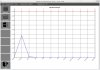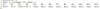# B4J QuestionRDC very long command Line

#### alienhunter

##### Active Member
Longtime User
hi ,
is there a way that i would be able to make this command more "friendly"

B4X:
``sql.select_month123=SELECT EXTRACT(YEAR FROM date) AS yr,SUM(CASE WHEN EXTRACT(MONTH FROM date) IN (?)THEN pointsreq END) AS Q1,SUM(CASE WHEN EXTRACT(MONTH FROM date) IN (?)THEN pointsreq END) AS Q2,SUM(CASE WHEN EXTRACT(MONTH FROM date) IN (?)THEN pointsreq END) AS Q3 FROM transactions GROUP BY EXTRACT(YEAR FROM date);``
this is only for 3 months and i have to do this for 12 ... i am out of screen's(2) widthexample :

B4X:
``````sql.select_month123=SELECT EXTRACT(YEAR FROM date) AS yr,SUM(CASE WHEN EXTRACT(MONTH FROM date) IN (?)THEN pointsreq END) AS Q1, & _
,SUM(CASE WHEN EXTRACT(MONTH FROM date) IN (?)THEN pointsreq END) AS Q1, &_
,SUM(CASE WHEN EXTRACT(MONTH FROM date) IN (?)THEN pointsreq END) AS Q1, &_
,SUM(CASE WHEN EXTRACT(MONTH FROM date) IN (?)THEN pointsreq END) AS Q1, &_
.... ...``````

thanks

Last edited:

#### alienhunter

##### Active Member
Longtime User
Thank you
it worked•Erel